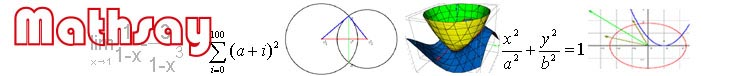Home

Support

Examples

Geometry

Trigonometry

Coordinate geometry

Function

Equation

Vector

3D geometry

3D plot

Limit & derivative

Math symbol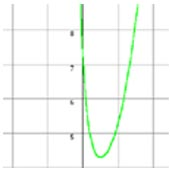f(x)=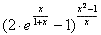. Show its limit, when x ->0.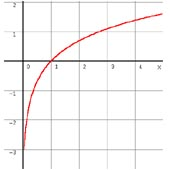Show the limit of 2lnx+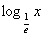,when x ->0.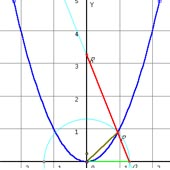Get line segment OQ in x axis and makes it equal to the length of chord OP of  curve y=x ^2which passes through O. Make line PQ. It intersects y axis in R. When P move to O, where will R go?.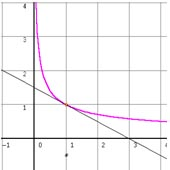f(x)=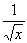. Find the slope of  graph of f(x) at point 1.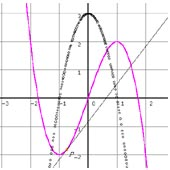Show the derivative of y=3x-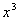.